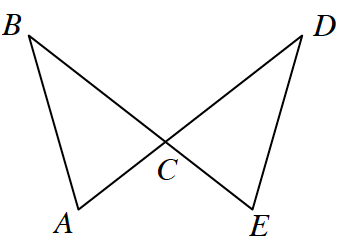### Home > CCG > Chapter 8 > Lesson 8.3.1 > Problem8-96

8-96.

Examine the diagram at right. Assume that $\overline {AD}$ and $\overline{BE}$ are line segments, and that $\overline{BC} \cong \overline{DC}$ and $\angle A \cong \angle E$ . Prove that $\overline{AB} \cong \overline{ED}$. Use the form of proof that you prefer (such as the flowchart or two-column proof format). Be sure to copy the diagram onto your paper and add any appropriate markings. Homework Help ✎

The triangles are congruent by $AAS\cong$.